×#### Thank you for registering.

One of our academic counsellors will contact you within 1 working day.

Click to Chat

1800-1023-196

+91-120-4616500

CART 0

• 0

MY CART (5)

Use Coupon: CART20 and get 20% off on all online Study Material

ITEM
DETAILS
MRP
DISCOUNT
FINAL PRICE
Total Price: Rs.

There are no items in this cart.
Continue Shopping• Complete JEE Main/Advanced Course and Test Series
• OFFERED PRICE: Rs. 15,900
• View Details

```Chapter 10: Congruent Triangles Exercise – 10.3

Question: 1

In two right triangles one side an acute angle of one are equal to the corresponding side and angle of the other. Prove that the triangles are congruent.

Solution:

Given that, in two right triangles one side and acute angle of one are equal to the corresponding side and angles of the other.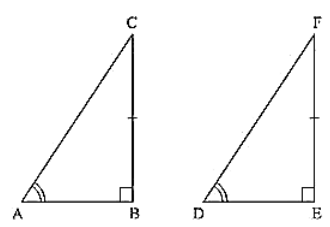We have to prove that the triangles are congruent.

Let us consider two right triangles such that

∠B = ∠C = 90° .... (i)

AB = DE .... (ii)

∠C = ∠F       From(iii)

Now observe the two triangles ABC and DEF

∠C = ∠F [From (iii)]

∠B = ∠E [From (i)]

and AB = DE   [From (ii)]

So, by AAS congruence criterion, we have ΔABC ≅ ΔDEF

Therefore, the two triangles are congruent

Hence proved

Question: 2

If the bisector of the exterior vertical angle of a triangle be parallel to the base. Show that the triangle is isosceles.

Solution:

Given that the bisector of the exterior vertical angle of a triangle is parallel to the base and we have to prove that the triangle is isosceles. Let ABC be a triangle such that AD is the angular bisector of exterior vertical angle EAC and AD ∥ BC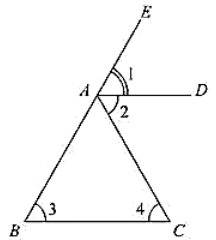Let ∠EAD = (i), ∠DAC = (ii), ∠ABC = (iii) and ∠ACB = (iv)

We have,

(i) = (ii)                       [AD is a bisector of ∠EAC]

(i) = (iii)                      [Corresponding angles]

and (ii) = (iv)             [alternative angle]

(iii) = (iv)

AB = AC

Since, in ΔABC, two sides AB and AC are equal we can say that ΔABC is isosceles triangle.

Question: 3

In an isosceles triangle, if the vertex angle is twice the sum of the base angles, calculate the angles of the triangle.

Solution:

Let ΔABC be isosceles such that AB = AC.

∠B = ∠C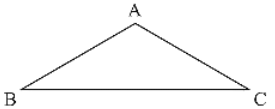Given that vertex angle A is twice the sum of the base angles B and C. i.e., ∠A= 2(∠B + ∠C)

∠A = 2(∠B + ∠B)

∠A = 2(2∠B)

∠A = 4(∠B)

Now, We know that sum of angles in a triangle = 180°

∠A + ∠B + ∠C =180°

4∠B + ∠B + ∠B = 180°

6∠B =180°

∠B = 30°

Since, ∠B = ∠C

∠B = ∠C = 30°

And ∠A = 4∠B

∠A = 4 × 30° = 120°

Therefore, angles of the given triangle are 120°, 30° and 30°.

= 428 and LB = LC]

Question: 4

PQR is a triangle in which PQ = PR and is any point on the side PQ. Through S, a line is drawn parallel to QR and intersecting PR at T. Prove that PS = PT.

Solution:

Given that PQR is a triangle such that PQ = PR ant S is any point on the side PQ and ST ∥ QR.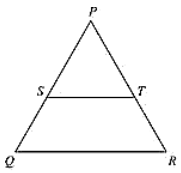To Prove,

PS = PT

Since, PQ = PR

PQR is an isosceles triangle.

∠Q = ∠R (or) ∠PQR = ∠PRQ

Now, ∠PST = ∠PQR and ∠PTS = ∠PRQ [Corresponding angles as ST parallel to QR]

Since, ∠PQR = ∠PRQ

∠PST = ∠PTS

Now, In ΔPST, ∠PST = ∠PTS

ΔPST is an isosceles triangle

Therefore, PS = PT

Question: 5

In a ΔABC, it is given that AB = AC and the bisectors of B and C intersect at O. If M is a point on BO produced, prove that ∠MOC = ∠ABC.

Solution:

Given that in ΔABC,

AB = AC and the bisector of ∠B and ∠C intersect at O. If M is a point on BO producedWe have to prove ∠MOC = ∠ABC

Since,

AB = AC

ABC is isosceles

∠B = ∠C (or)

∠ABC = ∠ACB

Now,

BO and CO are bisectors of ∠ ABC and ∠ACB respectively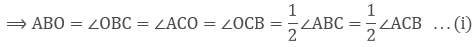We have, in Δ OBC

∠OBC + ∠OCB + ∠BOC = 180°  ... (ii)

And also

∠BOC + ∠COM = 180° ... (iii) [Straight angle]

Equating (ii) and (iii)

∠OBC + ∠OCB + ∠BOC = ∠BOC + ∠MOC

∠OBC + ∠OCB = ∠MOC [From (i)]

2∠OBC = ∠MOC [From (i)]∠ABC = ∠MOC

Therefore, ∠MOC = ∠ABC

Question: 6

P is a point on the bisector of an angle ABC. If the line through P parallel to AB meets BC at Q, prove that triangle BPQ is isosceles.

Solution:

Given that P is a point on the bisector of an angle ABC, and PQ ∥ AB.

We have to prove that ΔBPQ is isosceles.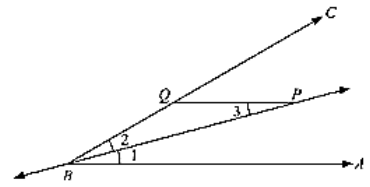Since,

BP is bisector of ∠ABC = ∠ABP = ∠PBC .... (i)

Now,

PQ ∥ AB

∠BPQ = ∠ABP ... (ii) [alternative angles]

From (i) and (ii), we get

∠BPQ = ∠PBC (or) ∠BPQ = ∠PBQ

Now,

In BPQ,

∠BPQ = ∠PBQ

ΔBPQ is an isosceles triangle.

Hence proved

Question: 7

Prove that each angle of an equilateral triangle is 60°.

Solution:

Given to prove each angle of an equilateral triangle is 60°.

Let us consider an equilateral triangle ABC.

Such that AB = BC = CA

Now, AB = BC

∠A = ∠C ... (i) [Opposite angles to equal sides are equal]

And BC = AC

∠B = ∠A ... (ii)

From (i) and (ii), we get

∠A = ∠B = ∠C  ... (iii)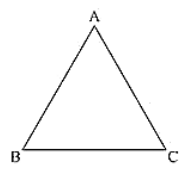We know that

Sum of angles in a triangle = 180

∠A + ∠B + ∠C = 180

∠A + ∠A + ∠A = 180

3∠A = 180

∠A = 60

∠A = ∠B = ∠C = 60

Hence, each angle of an equilateral triangle is 60°.

Question: 8

Angles ΔA, B, C of a triangle ABC are equal to each other. Prove that ABC is equilateral.

Solution:

Given that angles A, B, C of a triangle ABC equal to each other.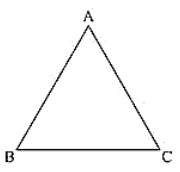We have to prove that ΔABC is equilateral

We have, ∠A = ∠B = ∠C

Now,

∠A = ∠B

BC = AC [opposite sides to equal angles are equal]

And ∠B = ∠C

AC = AB

From the above we get

AB = BC = AC

ΔABC is equilateral.

Question: 9

ABC is a triangle in which ∠B = 2∠C. D is a point on BC such that AD bisects ∠BAC and AB = CD. Prove that [∠BAC = 72.

Solution:

Given that in ABC, ∠B = 2 ∠C and D is a point on BC such that AD bisectors ∠BAC and AB = CD.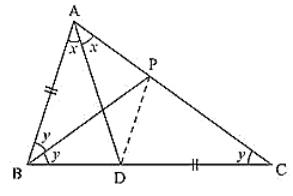We have to prove that ∠BAC = 72°

Now, draw the angular bisector of ∠ABC, which meets AC in P.

Join PD

Let C = ∠ACB = y

∠B = ∠ABC = 2∠C = 2y and also

∠BAC = 2x [AD is the bisector of ∠BAC]

Now, in ΔBPC,

∠CBP = y [BP is the bisector of ∠ABC]

∠PCB = y

∠CBP = ∠PCB = y [PC = BP]

Consider, ΔABP and ΔDCP, we have

ΔABP = ΔDCP = y

AB = DC                            [Given]

And PC = BP                     [From above]

So, by SAS congruence criterion, we have ΔABP ≅ ΔDCP

Now,

∠BAP = ∠CDF and AP = DP [Corresponding parts of congruent triangles are equal]

∠BAP = ∠CDP = 2

Consider, ΔAPD,

We have AP = DP

But ∠DAP = x

Now

In ΔABD.

From the above two equations, we get

2y + x = ∠ADP + ∠PDC

2y + x = x + 2x

2y = 2x

y = x (or) x = y

We know,

Sum of angles in a triangle = 180°

So, In ΔABC,

∠A + ∠B + ∠C =180°

2x + 2y + y = 180° [∠A = 2x, ∠B = 2y, ∠C = y]

2(y) + 3y =180° [x = y]

5y = 180°

y = 36°

Now, ∠A = ∠BAC = 2 × 36° = 72°

Question: 10

ABC is a right angled triangle in which ∠A = 90° and AB = AC. Find ∠B and ∠C.

Solution:

Given that ABC is a right angled triangle such that ∠A = 90° and AB = AC

Since, AB = AC

ΔABC is also isosceles.

Therefore, we can say that ΔABC is right angled isosceles triangle.

∠C = ∠B and ∠A = 90° ... (i)

Now, we have sum of angled in a triangle = 180°

∠A + ∠B + ∠C = 180°

90° + ∠B + ∠B = 180° [From (i)]

2∠B = 180° - 90°

∠B = 45°

Therefore, ∠B = ∠C = 45°
```### Course Features

• 728 Video Lectures
• Revision Notes
• Previous Year Papers
• Mind Map
• Study Planner
• NCERT Solutions
• Discussion Forum
• Test paper with Video Solution